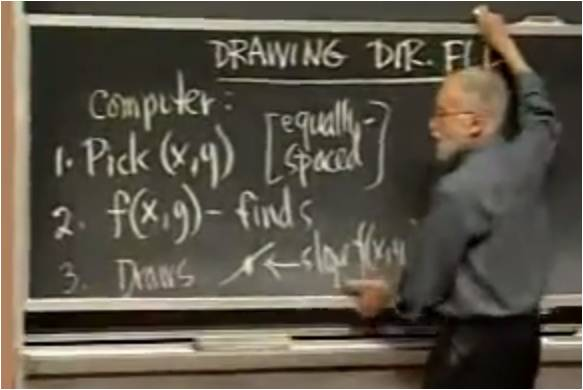# InfoCoBuild

## 18.03 Differential Equations

18.03 Differential Equations (Spring 2010, MIT OCW). Taught by Professor Arthur Mattuck, this course is a study of Ordinary Differential Equations (ODEs), including modeling physical systems. Differential Equations are the language in which the laws of nature are expressed. Understanding properties of solutions of differential equations is fundamental to much of contemporary science and engineering. Ordinary differential equations (ODEs) deal with functions of one variable, which can often be thought of as time. Topics covered in this course include: Solution of first-order ODEs by analytical, graphical and numerical methods; Linear ODEs; Undetermined coefficients and variation of parameters; Sinusoidal and exponential signals; Complex numbers and exponentials; Fourier series, periodic solutions; Delta functions, convolution, and Laplace transform methods; Matrix and first order linear systems; and Nonlinear autonomous systems. (from ocw.mit.edu)Lecture 01 - The Geometrical View of y'= f(x,y): Direction Fields, Integral Curves Lecture 02 - Euler's Numerical Method for y'=f(x,y) and its Generalizations Lecture 03 - Solving First-order Linear ODEs; Steady-state and Transient Solutions Lecture 04 - First-order Substitution Methods: Bernoulli and Homogeneous ODEs Lecture 05 - First-order Autonomous ODEs: Qualitative Methods, Applications Lecture 06 - Complex Numbers and Complex Exponentials Lecture 07 - First-order Linear with Constant Coefficients Lecture 08 - Continuation; Applications to Temperature, RC-circuit, Growth Models Lecture 09 - Solving Second-order Linear ODEs with Constant Coefficients Lecture 10 - Continuation: Complex Characteristic Roots; Undamped and Damped Oscillations Lecture 11 - Theory of General Second-order Linear Homogeneous ODEs Lecture 12 - Continuation: General Theory for Inhomogeneous ODEs Lecture 13 - Finding Particular Solutions to Inhomogeneous ODEs Lecture 14 - Interpretation of the Exceptional Case: Resonance Lecture 15 - Introduction to Fourier Series Lecture 16 - Continuation: More General Periods; Even and Odd Functions; Periodic Extension Lecture 17 - Finding Particular Solutions via Fourier Series; Resonant Terms; Hearing Musical Sounds Lecture 18 Lecture 19 - Introduction to the Laplace Transform Lecture 20 - Derivative Formulas Lecture 21 - Convolution Formula Lecture 22 - Using Laplace Transform to Solve ODEs with Discontinuous Inputs Lecture 23 - Use with Impulse Inputs; Dirac Delta Function, Weight and Transfer Functions Lecture 24 - Introduction to First-order Systems of ODEs Lecture 25 - Homogeneous Linear Systems with Constant Coefficients Lecture 26 - Continuation: Repeated Real Eigenvalues, Complex Eigenvalues Lecture 27 - Sketching Solutions of 2x2 Homogeneous Linear System with Constant Coefficients Lecture 28 - Matrix Methods for Inhomogeneous Systems Lecture 29 - Matrix Exponentials; Application to Solving Systems Lecture 30 - Decoupling Linear Systems with Constant Coefficients Lecture 31 - Non-linear Autonomous Systems Lecture 32 - Limit Cycles: Existence and Nonexistence Criteria Lecture 33 - Relation Between Non-linear Systems and First-order ODEs

 References 18.03 Differential Equations Instructors: Prof. Arthur Mattuck and Prof. Haynes Miller. Lecture Notes. Exams and Solutions. Subtitles/Transcript. Assignments and Solutions. Differential Equations are the language in which the laws of nature are expressed.